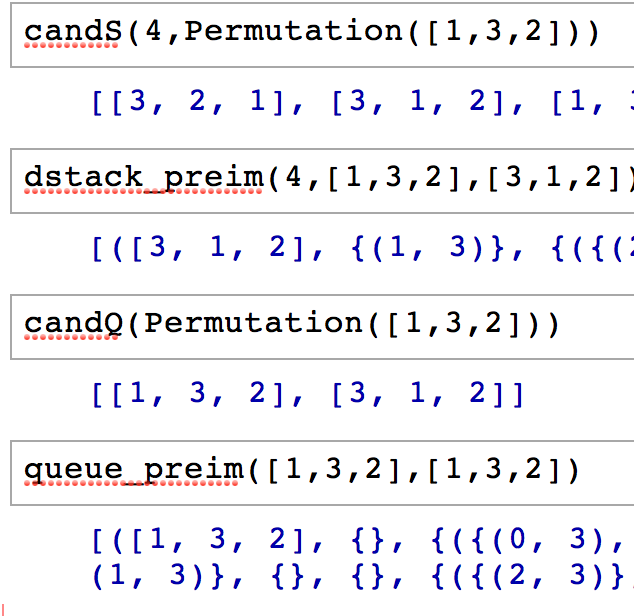### Algorithms computing the preimages of sorting operators

The file preim.sage contains algorithms developed by myself and Hjalti Magnusson for computing the preimage of any pattern class under two sorting operators: 1) A stack of depth d (you can put d equal to infinity); and 2) A queue. These are implemented in the computer algebra system Sage. What follow are instructions for using the algorithm. Please contact me via email if you find any bugs, want any clarifications or to suggest new features.#### The preimage for a stack of depth d

The instructions below assume you have Sage on your machine and have downloaded the file preim.sage and put it in you home directory. Start Sage and then type `load '~/preim.sage'` into the first cell. We now want to describe the permutations that avoid 132 after passing through a stack of depth 4. The following code will find all classical patterns that could possibly be shaded marked and decorated to become 132 after sorting.

```'''
Finding the candidates for the pattern target for a stack of depth d
'''

d      = 4
target = Permutation([1,3,2])

candS(d,target)
```

The output is `[[3, 2, 1], [3, 1, 2], [1, 3, 2]]`. For each one of these candidates we need to check how they must be shaded, marked and decorated. First we look at the candidate 321:

```'''
For each candidate we find how it must be shaded, marked and decorated
to become target after sorting
'''

d         = 4
target    = Permutation([1,3,2])
candidate = Permutation([3,2,1])

dstack_preim(d,target,candidate)
```

The output is an empty list, meaning there is no way to shade, mark and decorate 321 to become 132 after sorting. Now consider the next candidate, 312:

```'''
For each candidate we find how it must be shaded, marked and decorated
to become target after sorting
'''

d         = 4
target    = Permutation([1,3,2])
candidate = Permutation([3,1,2])

dstack_preim(d,target,candidate)
```

The output is now a list containing one element: `([3, 1, 2], {(1, 3)}, {({(2, 3)}, 1)}, {({(0, 3)}, 3)}, {})`. This means that we should shade the box (1,3), mark the box (2,3) with "1" and the square (0,3) must be decorated to avoid the pattern V3, defined on page 17 in Hjalti's thesis. Finally consider the last candidate, 132:

```'''
For each candidate we find how it must be shaded, marked and decorated
to become target after sorting
'''

d         = 4
target    = Permutation([1,3,2])
candidate = Permutation([1,3,2])

dstack_preim(d,target,candidate)
```

The output is a list containing two elements:

• `([1, 3, 2], {}, {({(2, 3)}, 1)}, {}, {})`. This means that we should shade the box mark the box (2,3) with "1".
• `([1, 3, 2], {(2, 3)}, {}, {},{({(0, 3), (1, 3)}, 3)})`. This pattern has the box (2,3) shaded and decorated to contain the pattern V3 in the box (1,3).

#### The preimage for a queue

We now want to describe the permutations that avoid 132 after passing through a queue. The following code will find all classical patterns that could possibly be shaded marked and decorated to become 132 after sorting.

```'''
Finding the candidates for the pattern target, for a queue
'''

target = Permutation([1,3,2])

candQ(target)
```

The output is `[[1, 3, 2], [3, 1, 2]]`. For each one of these candidates we need to check how they must be shaded, marked and decorated. First we look at the candidate 132:

```'''
For each candidate we find how it must be shaded, marked and decorated
to become target after sorting
'''

target    = Permutation([1,3,2])
candidate = Permutation([1,3,2])

queue_preim(target,candidate)
```

The output is a list containing two elements:

• `([1, 3, 2], {}, {({(0, 3), (1, 3)}, 1)}, {}, {})`. This means that we should shade the box mark the region consisting of (0,3) and (1,3) with "1".
• `([1, 3, 2], {(0, 3),(1, 3)}, {}, {}, {({(2, 3)}, [2, 1])})`. This pattern has the boxes (0,3) and (1,3) shaded and decorated to contain the pattern 21 in the box (2,3).

If we run the same code for the candidate 312 we will get an empty list as output, meaning that 312 can not be shaded, marked and decorated to become 132 after being sorted with a queue.